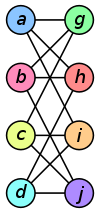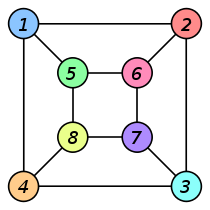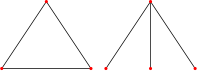# Graph isomorphism

Graph isomorphism

In graph theory, an isomorphism of graphs G and H is a bijection between the vertex sets of G and H$f \colon V(G) \to V(H) \,\!$

such that any two vertices u and v of G are adjacent in G if and only if ƒ(u) and ƒ(v) are adjacent in H. This kind of bijection is commonly called "edge-preserving bijection", in accordance with the general notion of isomorphism being a structure-preserving bijection.

In the above definition, graphs are understood to be undirected non-labeled non-weighted graphs. However, the notion of isomorphism may be applied to all other variants of the notion of graph, by adding the requirements to preserve the corresponding additional elements of structure: arc directions, edge weights, etc., with the following exception. When spoken about graph labeling with unique labels, commonly taken from the integer range 1,...,n, where n is the number of the vertices of the graph, two labeled graphs are said to be isomorphic if the corresponding underlying unlabeled graphs are isomorphic.

If an isomorphism exists between two graphs, then the graphs are called isomorphic and we write$G\simeq H$. In the case when the bijection is a mapping of a graph onto itself, i.e., when G and H are one and the same graph, the bijection is called an automorphism of G.

The graph isomorphism is an equivalence relation on graphs and as such it partitions the class of all graphs into equivalence classes. A set of graphs isomorphic to each other is called an isomorphism class of graphs.

## Example

The two graphs shown below are isomorphic, despite their different looking drawings.

Graph G Graph H An isomorphism
between G and Hƒ(a) = 1

ƒ(b) = 6

ƒ(c) = 8

ƒ(d) = 3

ƒ(g) = 5

ƒ(h) = 2

ƒ(i) = 4

ƒ(j) = 7

## Motivation

The formal notion of "isomorphism", e.g., of "graph isomorphism", captures the informal notion that some objects have "the same structure" if one ignores individual distinctions of "atomic" components of objects in question, see the example above. Whenever individuality of "atomic" components (vertices and edges, for graphs) is important for correct representation of whatever is modeled by graphs, the model is refined by imposing additional restrictions on the structure, and other mathematical objects are used: digraphs, labeled graphs, colored graphs, rooted trees and so on. The isomorphism relation may also be defined for all these generalizations of graphs: the isomorphism bijection must preserve the elements of structure which define the object type in question: arcs, labels, vertex/edge colors, the root of the rooted tree, etc.

The notion of "graph isomorphism" allows us to distinguish graph properties inherent to the structures of graphs themselves from properties associated with graph representations: graph drawings, data structures for graphs, graph labelings, etc. For example, if a graph has exactly one cycle, then all graphs in its isomorphism class also have exactly one cycle. On the other hand, in the common case when the vertices of a graph are (represented by) the integers 1, 2,... N, then the expression$\sum_{v \in V(G)} v\cdot\text{deg }v$

may be different for two isomorphic graphs.

## Recognition of graph isomorphism

### Whitney theoremThe exception of Whitney's theorem: these two graphs are not isomorphic but have isomorphic line graphs.

The Whitney graph isomorphism theorem, shown by H. Whitney, states that two connected graphs are isomorphic if and only if their line graphs are isomorphic, with a single exception: K3, the complete graph on three vertices, and the complete bipartite graph K1,3, which are not isomorphic but both have K3 as their line graph. The Whitney graph theorem can be extended to hypergraphs.

### Algorithmic approach

While graph isomorphism may be studied in a classical mathematical way, as exemplified by the Whitney theorem, it is recognized that it is a problem to be tackled with an algorithmic approach. The computational problem of determining whether two finite graphs are isomorphic is called the graph isomorphism problem.

Its practical applications include primarily cheminformatics, mathematical chemistry (identification of chemical compounds), and electronic design automation (verification of equivalence of various representations of the design of an electronic circuit).

Curiously, it is also one of only a few problems in computational complexity theory belonging to NP, but not known to belong to either of its well-known (and, if P ≠ NP, disjoint) subsets: P and NP-complete. It is one of only two, out of 12 total, problems listed in Garey & Johnson (1979) whose complexity remains unresolved. That is, it has not been proven to be included in, nor excluded from, P or NP-complete. Its generalization, the subgraph isomorphism problem, is known to be NP-complete.

The main areas of research for the problem is design of fast algorithms, both for the general problem and for special classes of graphs, and theoretical investigations of its computational complexity.

Wikimedia Foundation. 2010.

### См. также в других словарях:

• Graph automorphism — In graph theoretical mathematics, an automorphism of a graph is a form of symmetry in which the graph is mapped onto itself while preserving the edge vertex connectivity.Formally, an automorphism of a graph G = ( V , E ) is a permutation sigma;… …   Wikipedia

• Isomorphism — In abstract algebra, an isomorphism (Greek: ἴσος isos equal , and μορφή morphe shape ) is a bijective map f such that both f and its inverse f −1 are homomorphisms, i.e., structure preserving mappings.In the more general setting of category… …   Wikipedia

• Graph homomorphism — Not to be confused with graph homeomorphism. In the mathematical field of graph theory a graph homomorphism is a mapping between two graphs that respects their structure. More concretely it maps adjacent vertices to adjacent vertices. Contents 1… …   Wikipedia

• Graph coloring — A proper vertex coloring of the Petersen graph with 3 colors, the minimum number possible. In graph theory, graph coloring is a special case of graph labeling; it is an assignment of labels traditionally called colors to elements of a graph… …   Wikipedia

• Graph property — In graph theory a graph property is any inherently graph theoretical property of graphs (formal definitions follow), distinguished from properties of graphs described in terms of various graph representations: graph drawings, data structures for… …   Wikipedia

• Isomorphism problem — The isomorphism problem in mathematics may refer to* The graph isomorphism problem * The group isomorphism problem …   Wikipedia

• Graph rewriting — In graph theory, graph rewriting is a system of rewriting for graphs, i.e. a set of graph rewrite rules of the form p: L ightarrow R, with L being called pattern graph (or left hand side) and R being called replacement graph (or right hand side… …   Wikipedia

• Graph theory — In mathematics and computer science, graph theory is the study of graphs : mathematical structures used to model pairwise relations between objects from a certain collection. A graph in this context refers to a collection of vertices or nodes and …   Wikipedia

• Rado graph — The Rado graph, as numbered by Rado (1964). In the mathematical field of graph theory, the Rado graph, also known as the random graph or the Erdős–Renyi graph, is the unique (up to isomorphism) countable graph R such that for any finite graph G… …   Wikipedia

• Skew-symmetric graph — In graph theory, a branch of mathematics, a skew symmetric graph is a directed graph that is isomorphic to its own transpose graph, the graph formed by reversing all of its edges. The isomorphism is required to be an involution without any fixed… …   Wikipedia

### Поделиться ссылкой на выделенное

##### Прямая ссылка:
Нажмите правой клавишей мыши и выберите «Копировать ссылку»